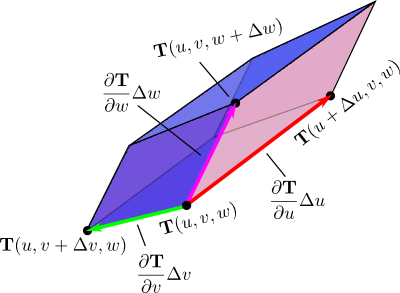# Math Insight

### Image: Parallelepiped approximation underlying volume transformation calculationThe change of variables function $\cvarf$ transforms a small box to a curvy region in $xyz$ space. But, since we view the dimensions $\Delta\cvarfv \times \Delta\cvarsv \times \Delta\cvartv$ as being small, we can approximate the image of the box as the shown parallelepiped. Since the image of the box is approximated as a parallelepiped, we can easily calculate its volume using the scalar triple product.

Image file: change_variable_volume_parallelepiped.png

Source image file: change_variable_volume_parallelepiped.svg
Source image type: Inkscape SVG